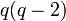Element structure of general affine group of degree one over a finite field

Contents

This article gives specific information, namely, element structure, about a family of groups, namely: general affine group of degree one.
View element structure of group families | View other specific information about general affine group of degree one

This article describes the element structure of the general affine group of degree one over a finite field. We denote the field size by the letter$q$ and the characteristic of the field by the letter$p$. Note that$q$ is a prime power with underlying prime$p$.

Summary

Item Value
number of conjugacy classes$q$
equals number of irreducible representations, see also linear representation theory of general affine group of degree one over a finite field
order$q(q - 1)$
exponent$p(q - 1)$
conjugacy class size statistics size 1 (1 class), size$q - 1$ (1 class), size$q$ ($q - 2$ classes)

Conjugacy class structure

All the elements of this group are of the form:$x \mapsto ax + v, a \in \mathbb{F}_q^\ast, v \in \mathbb{F}_q$

Nature of conjugacy class Size of conjugacy class Number of such conjugacy classes Total number of elements$a = 1, v = 0$ 1 1 1$a = 1, v \ne 0$ (conjugacy class is independent of choice of$v$)$q - 1$ 1$q - 1$$a \ne 1$ (conjugacy class is determined completely by choice of$a$ and is independent of choice of$v$; in other words, each conjugacy class is a coset of the subgroup of translations)$q$$q - 2$$q(q - 2)$
Total (--) --$q$$q(q - 1)$